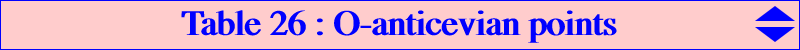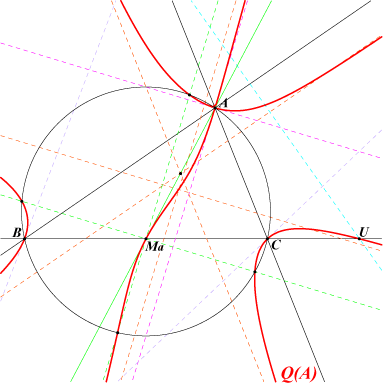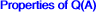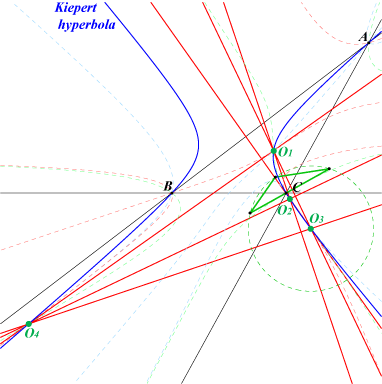Let P be a point and PaPbPc its anticevian triangle. We say that P is a O-anticevian point if and only if P is the circumcenter of PaPbPc. This is generalized in : Table 28 : cevian and anticevian points. P must lie on the perpendicular bisector of PbPc hence P must lie on the nodal quartic Q(A) with equation : a^2(y-z)(x+y+z)yz - b^2z[(x+y)(y-z)^2+x^2z] + c^2y[(x+z)(y-z)^2+x^2y] = 0.A is a node and the nodal tangents at A are the bisectors of ABC. the tangents at B and C to Q(A) meet at a point on the line AO. Q(A) contains Ma, midpoint of BC, where the tangent is the median AG and U, foot of the orthic axis on BC. Q(A) has four real asymptotes : two are parallel to the sidelines AB, AC and two are parallel to the bisectors at A. Q(A) meets the circumcircle at A (double), B, C and four points on the parallels at Ma to the bisectors at A.Similarly, the quartics Q(B) and Q(C) are defined and it is clear that any point on two of the quartics must lie on the third one. Q(B) and Q(C) have 16 common points namely : A, B, C counting for 4, the infinite point of BC counting for 3 since the two cubics have the same asymptote at this point, two always imaginary points on the line MbMc counting for 4 sinces the tangents at these points are the same and pass through A. These 12 points do not give a proper anticevian triangle and this leaves four (not always real) points which we call the O-anticevian points (green points in the triangle 6-9-13) and denote Oi.Now, for any point R=p:q:r, we denote by Q(R) the quartic Q(R) = p Q(A) + q Q(B) + r Q(C) which obviously contains the points Oi. The most interesting case is Q(G) since the quartic decomposes into the Steiner inellipse (which cannot contain the Oi) and the Kiepert hyperbola. Hence we have the theorem : The O-anticevian points lie on the Kiepert hyperbola. It is a known fact that a parametrization of the Kiepert hyperbola is given by : P(t) = 1/(SA + S t) : 1/(SB + S t) : 1/(SC + S t), where S is twice the area of ABC and t the cotangent of the base angle of the isosceles triangles erected on the sides of ABC. A tedious computation shows that P(t) is a O-anticevian point if and only if t is a solution of the equation : 1 + 2(cotW + 4 cotA cotB cotC) t + 8 t^2 + 2 cotW t^3 - t^4 = 0 (W is the Brocard angle). A numerical investigation in the triangle 6-9-13 gives the SEARCH numbers of the four points namely : -6.8774950107, -1.8718980298, -0.31044570945, 2.2240565054.The O-anticevian points form an orthocentric system. Indeed, every conic passing through them is a rectangular hyperbola. Jean-Pierre Ehrmann has found that (H) passing through X(6), X(143), X(265), X(389), X(1209) contains the points. Its asymptotes are parallel to those of the Jerabek hyperbola. It follows that two of these Oi points are always real since the pencil of rectangular hyperbolas contains hyperbolas not having the same infinite points. The locus of their centers is naturally a circle which contains X(115) and no other known center.The figure shows one of the Oi points with its anticevian triangle and circle.A property of the Kiepert hyperbola and other quartics through the Oi points For any P in the plane, let O(P) be the circumcenter of the anticevian triangle of P. The points P, O(P) and H are collinear if and only if P lies on the Kiepert hyperbola (together with the polar circle : this circle is a diagonal conic hence the vertices of the anticevian triangle of any P on the circle also lie on the circle which means that O(P) = H). More generally, for any given point Z, the points P, O(P) and Z are collinear if and only if P lies on a circum-quartic Q'(Z) which obviously contains the Oi points and Z. In particular, Q'(A) is the locus of P such that A, P, O(P) are collinear. The figure below shows Q'(O) with only two real points Oi.Points on Q'(O) Oi points I, G, O, excenters Ma, Mb, Mc midpoints of ABC cevians of X(523) -a^2 : 2SA : 2SA on the median AG (harmonic conjugate of A4 with respect to A and Ma, A4 being the A-vertex of the fourth Brocard triangle) (b+c)(2SA-bc) : b(2SA+bc) : c(2SA+bc) on the internal bisector at A (b-c)(2SA+bc) : b(2SA-bc) : -c(2SA-bc) on the external bisector at A (the line through these two points is perpendicular to the Euler line) analogous points on the other medians and bisectorsOther curves These Oi points lie on the sextic Q057.